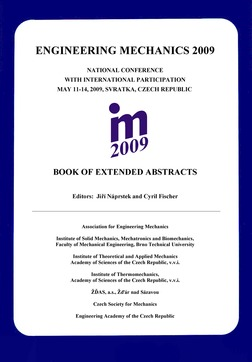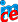# Engineering Mechanics

## International Conference

### Proceedings Vol. 15 (2009)### ENGINEERING MECHANICS 2009

NATIONAL CONFERENCE WITH INTERNATIONAL PARTICIPATION
May 11 – 14, 2009, Svratka, Czech Republic
;
Editors: Jiří Náprstek and Cyril Fischer

Copyright © 2009 Institute of Theoretical and Applied Mechanics, Academy of Sciences of the Czech Republic, v.v.i., Prague

ISBN 978-80-86246-35-2 (printed, Extended Abstracts)
ISSN 1805-8248 (printed)
ISSN 1805-8256 (electronic)

list of papers scientific commitee

Is the logarithmic time derivative simply the Zaremba-Jaumann derivative?

The paper raises a question whether the logarithmic time derivative, expressed in speciﬁc coordinate system, is the Zaremba-Jaumann time derivative, and if not why. In fact, it has been already proved that the Z-J derivative represents the geometrically consistent linearization of tensor ﬁelds in terms of the covariant derivative in the space of right Cauchy-Green deformation tensors C. This is the space of symmetric, positive-deﬁnite 3×3 matrices of real numbers Sym+ (3, R) ∼ = + GL (3, R)/SO(3, R), which has a natural geometry of a Riemannian (globally) symmetric space of constant curvature, with the covariant derivative based on its Riemannian metric. Since in this geometry matrix exponentials stand for geodesics (that is a generalization of straight lines), the logarithmic strain log(C) can be interpreted simply as the change of coordinates in Sym+ (3, R), called the normal coordinates. There are some indications that the Z-J time derivative expressed in this normal coordinate system might be the logarithmic time derivative.

back to list of papers

Text and facts may be copied and used freely, but credit should be given to these Proceedings.

All papers were reviewed by members of the scientific committee.Powered by Imce 3.13  © 2020, Pavel Formánek, Institute of Thermomechanics AS CR, v.v.i. [generated: 0.0254s]